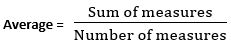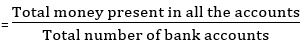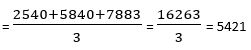## LetsPlayMaths.Com

WELCOME TO THE WORLD OF MATHEMATICS

# Class 5 Average

Average

Average Worksheet

## Average

Average is a single number which represents a value of group of numbers.

Average mark of all students is 75%, which means some of the students might have secured more than 75% and some students might have secured less than 75%.
Average of a group of numbers is calculated using following formula.Example 1. John scored 85 marks in English, 96 in Mathematics, 92 in science and 82 in history. What is John’s average score?

Solution.So, John scored an average 88.75 mark in his exam.

Example 2. Bob has Rs. 2540, Ryan has Rs. 5840, and John has Rs. 7883 in their respective bank accounts. Then, what is the average money kept in their bank accounts?

Solution.Average money in bank accountsSo, the average money kept in the account is Rs. 5421.

## Class-5 Average Worksheet

Average Worksheet - 1

Average Worksheet - 2